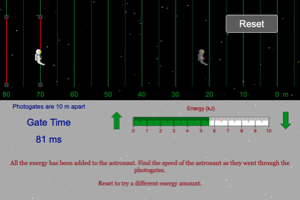Programs for Programs for Work, Energy and Power
Below are all the programs that might be used with this topic. Click on "See Resources" to see if there are any student directions, lab sheets or other materials that have been created for this program.
Energy Loss on Bounce Guided Lab

Lab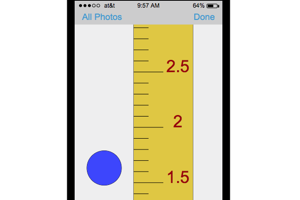Energy Loss on Bounce

Lab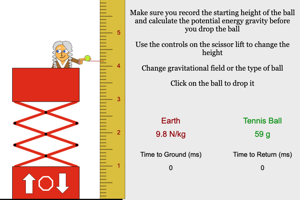Work to PEg Lab

Lab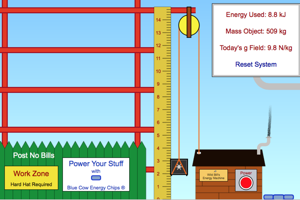Work to PEe Lab

Lab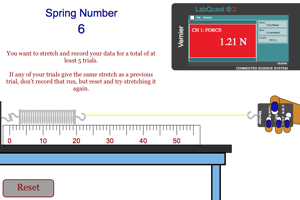Speed from Work Graph

Homework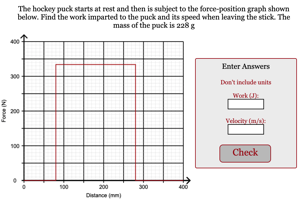Kinetic Energy of a Curling Stone

Homework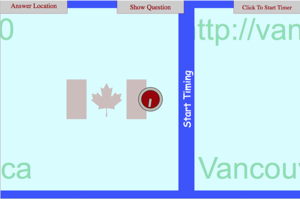Work Done from Horizontal Force with No Friction

Homework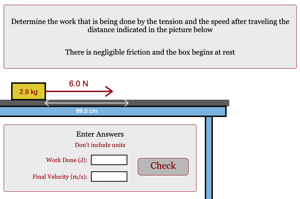Work Done from Force On Angle with No Friction

Homework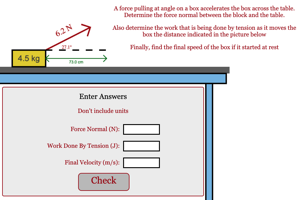Kinetic Energy Lab

Lab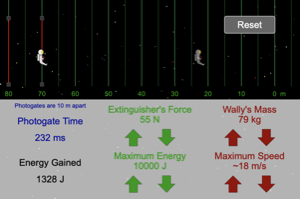Kinetic Energy with Predictions Lab

Lab